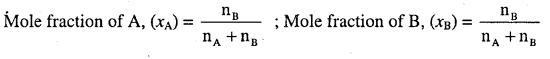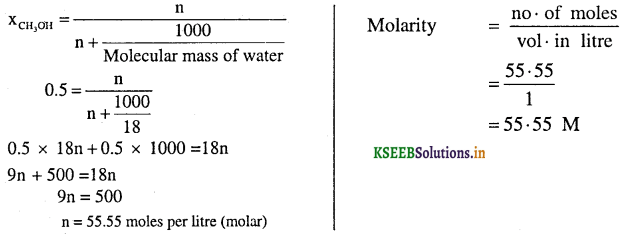# 1st PUC Chemistry Question Bank Chapter 1 Some Basic Concepts of Chemistry

Students can Download Chemistry Chapter 1 Some Basic Concepts of Chemistry Questions and Answers, Notes Pdf, 1st PUC Chemistry Question Bank with Answers helps you to revise the complete Karnataka State Board Syllabus and to clear all their doubts, score well in final exams.

## Karnataka 1st PUC Chemistry Question Bank Chapter 1 Some Basic Concepts of Chemistry

Question 1.
What is chemistry?
Chemistry is a branch of science which deals with the study of composition, structure and properties of  matter.

Question 2.
Name important branches of chemistry.
(a) Physical chemistry
(b) Organic chemistry
(c) Inorganic chemistry
(d) Biochemistry
(e) Analytical chemistry etc.

Question 3.
What is matter?
Any thing which has mass and occupies space is called matter.Question 4.
What are the basic constituents of matter?
Atoms and molecules.

Importance of Chemistry

Question 1.
Give any four importance of chemistry.

1. Chemistry plays an important role in providing food, cloth and shelter.
2. Many life saving drugs such as cis platin, taxol (both are used in cancer therapy) and AZT (Azidothymidine; used in the treatment of AIDS victims).
3. In the manufacture fertilizers, insecticides and pesticides.
4. In controlling pollution, industrial waste etc.
5. Large scale production of chemicals by using enzymes in biochemical processes.

Question 2.
Name the environmentally hazardous refrigerant responsible for ozone depletion in the stratosphere.
Chlorofluoro carbons (CFC)

Question 3.
Name the two green house gases.
Methane, CO2 etc.

Nature of Matter

Question 1.
Name the types of matter.
Matter is of three types- solids, liquids and gases.

Question 2.
Give two general characteristics of solids.

1. In solids, the particles are held very close to each other and there is no freedom of movement.
2. They have definite shape and definite volume.

Question 3.
Give two general characteristics of liquids.

1. In liquids, the particles are close to each other but they can move around.
2. They have definite volume but do not have definite shape.Question 4.
Give two general characteristics of gases.

1. In gases, the particles are far apart and their movement is easy and fast.
2. They do not have definite volume and definite shape.
3. They completely occupy the container in which they are placed.
Note: These three physical states of matter are interconvertible depending on the temperature and pressure.
Solid ⇌ Liquid ⇌ Gas

Question 5.
Give the schematic classification of matter.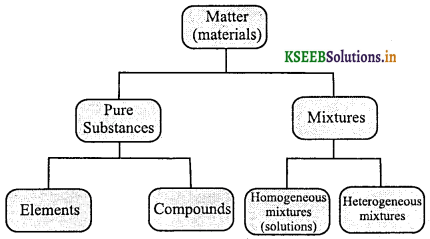Question 6.
What is mixture? Given an example.
Mixture is a homogeneous or heterogeneous forms of matter and composed of variable proportions of different components. Example: sugar solution in water.

Question 7.
What is homogeneous mixture? Give an example.
Mixture of two or more components forming a single phase and having uniform throughout the composition is called homogenous mixture. Example: sugar solution in water.

Question 8.
What is heterogeneous mixture? Give an example.
Mixture of two or more components forming many phases and does not have uniform composition throughout is called heterogeneous mixture. Example: Mixture of water and ether.

Question 9.
What are pure substances?
Pure substance is a form of matter with definite and constant composition with definite chemical properties.

Question 10.
Can pure substances be separated by physical methods? If not, mention the method of separation.
No. By chemical methods only, they can be separated.Question 11.
Name the types of pure substances.
Two types

1. Elements
2. Compounds

Question 12.
Give any two differences between pure substances and mixture.

 Sl. No. Pure substances Mixture 1. They have fixed composition They donot have fixed composition 2. The constituents of pure substances cannot be separated by physical methods. They can be separated by physical methods

Question 13.
What is element?
Element is a form of pure substance made up of same kind of particles (atoms).

Question 14.
Name the types of particles present in elements? Give examples.
One type of particles – atoms or molecules.
Examples:

1. Copper is made up of atoms.
2. Hydrogen, nitrogen and oxygen gases are molecules in which two atoms combine to give molecules.

Question 15.
What is compound?
Compound is a form of pure substance made up of different kind of particles (elements or molecules).

Question 16.
How are compounds formed? Give examples.
When two or more atoms of different elements combine, compounds are obtained.
Examples: H2O, NH3, CO2 etc.

Properties of Matter and their Measurement

Question 1.
Name the types of properties of matter?
Two types
(i) Physical properties
(ii) Chemical properties

Question 2.
What are physical properties of substances? Give examples.
The properties which can be measured or observed without changing the identity or the composition of the substance are called physical properties. Examples: Colour, odour, melting point etc.

Question 3.
What are chemical properties of substances? Give examples.
The properties which can be observed and measured only on allowing the substances to undergo change in their identity and composition are called chemical properties.
Examples: Acidity, basicity, combustibility, etc.Question 4.
How many basic physical quantities are there in SI unit system?
Seven

Question 5.
Name the seven basic physical quantities, mention their units and symbols according to SI unit system.

 Physical quantity Name of the unit Symbol Mass kilogram kg Length metre m Time second s Temperature kelvin K Electric current ampere A Luminous intensity candela cd Amount of   substance mole mol

Question 6.
Give the definitions of fundamental physical quantities.

• Meter: The distance equal to 1, 650,763.73 wavelengths in vacuum of the orange-red line of the spectrum of krypton – 86 in vaccum.
• Kilogram: The mass of a cylinder of platinum-iridium alloy kept by the International Bureau of Weights and Measures at Paris.
• Second: The duration of 9, 192, 631,770 cycles of the radiation associated with a specified transition of caesium-133 atom.
• Ampere: The current that when flowing through each of two long parallel wires separated by 1-meter of free ‘ space, results in a force between the wires of 2 x 10-7 newton per meter of length.
• Kelvin: The fraction 1 / 273.16 of the temperature difference between absolute zero and triple point of water.
• Mole: The amount of a substance that contains as many entities as there are atoms in exactly 0.012 kilograms of carbon – 12.
• Candela: The luminous intensity of 1 / 600,000 of a square meter of a black body at the temperature of freezing platinum (2045 K)

Question 7.
Write the multiples and prefixes used in the SI system.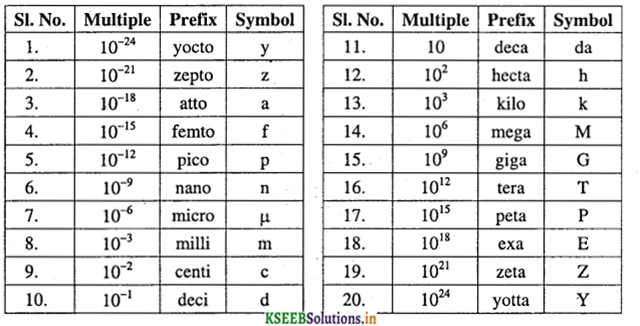\Question 8.
What is mass of a substance?
The amount of matter present in a substance is called mass.

Question 9.
What is weight of a substance?
The force excreted by gravity on an object is called weight.

Question 10.
What is the difference between mass and weight of a substance?

 Mass Weight 1. Mass of a substance is constant 1. Weight of a substance varies from place to place due to change in gravity. 2. Mass is scalar quantity 2. Weight is vector quantity

Question 11.
What is the SI unit of volume?
m3
Note: In chemistry laboratories smaller volumes are measured in cm3 or dm3 also.
1L = 1000 mL, 1000 cm3 = 1dm3, cm3 = mL, dm3 = L.

Question 12.
What is the SI unit of density?
kg m-3
Note: CGS unit (Non SI unit) of density is gcm-3

Question 13.
Name three common scales to measure temperature.

• Degree Celsius (°C)
• Degree Fahrenheit (°F)
• Kelvin (K)

Question 14.
Write the relation between °F and °C.
°F = $$\frac { 9 }{ 5 }$$ (°C) + 32

Question 15.
Convert 37°C to °F.
°F = $$\frac { 9 }{ 5 }$$(°C) + 32
= $$\frac { 9 }{ 5 }$$ × 37 + 32 = 98.6 °F

Question 16.
Convert 100° F into °C
°F = × (°C) + 32
100 = $$\frac { 9 }{ 5 }$$(°C)-32 ; $$\frac { 9 }{ 5 }$$(°C) = 100 – 32
$$\frac { 9 }{ 5 }$$(°C) = 68 5
(°C) = $$\frac{68 \times 5}{9}$$ = 37777°CQuestion 17.
Write the relation between °C and Kelvin (K).
K = °C + 273.15

Question 18.
Convert 27° C into Kelvin.
K = °C + 273.15
= 27 + 273.15 = 300.15 K

Question 19.
Convert 373 K into °C.
K = °C + 273.15
373 = °C + 273.15
°C = 373 – 273.15
= 99.85 °C

Uncertainty in Measurements

Question 1.
What is scientific notation?
It is an exponential notation of writing too large and too small numbers in the form N × 10n where n is an exponential having positive or negative values and N is the number which varies between 1 to 10.

Question 2.
Express the number 232.508 in scientific notation.
2.32508 × 102

Question 3.
Express number 0.00016 in scientific notation.
1.6 × 10-4

Question 4.
Express the following in the scientific notation.
(i) 0.0048
(ii) 234000
(iii) 8008
(iv) 500.0
(v) 6.0012
(i) 4.8 × 10-3
(ii) 2.34 × 105
(iii) 8.008 × 103
(iv) 5 × 102
(v) 6.0012

Question 5.
Define precision and accuracy.
Precision is the closeness of various measurements for the same quantity.
Accuracy is the agreement of a particular value to the true value of the result.

Question 6.
Four students determined the volume of a glass container in three separate times (see table below). The true volume of the container is 24.20 mL. Give the explanation of the student’s results in terms of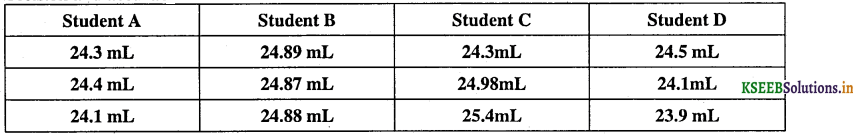1. Student A results are most precise and accurate because the results are very close to each other and also close to the true value.

2. Student B results are the most precise but not accurate because these results are very close to each other but not near to true value.

3. Student C results are neither precise nor accurate because these results are not close to each other or near to true volume.

4. Student D results are accurate because average of these values are near to true value but not precise because the values are not close to each other.Question 7.
What are significant figures? Give the general rules for determining the number of significant figures?
Significant figures are meaningful digits which are known with certainty.
Rules to assign significant figures

• All non-zero digits are significant.
• Zeros preceding to first non zero digit (leading zeros) are not significant.
• Zeros between two non-zero digits are significant.
• Zeros at the end or right of a number (trailing zeros) are significant provided they are on the right side of the decimal point.
• In numbers written in scientific notation, all digits are significant.

Question 8.
How many significant figures are present in the following:
(i) 0.0025
Two

(ii) 208
Three

(iii) 5005
Four

(iv) 126,000
Three

(v) 500.0
Four

(vi) 2.0034
Five

Question 9.
Give the rules for rounding of the numbers.

• If the rightmost digit to be removed is more than 5, the preceding number is increased by one.
• If the rightmost digit to be removed is less than 5, the preceding number is not changed.
• If the rightmost digit to be removed is 5, the preceding number is not changed if it is an even number but it is increased by one if it is an odd number.

For example, If 6.35 is rounded by removing 5, we have to increase 3 to 4 giving 6.4 as the result because preceding number is odd. However, if 6.25 is to be rounded off, it is rounded off to 6.2 because preceding number is even.

Question 10.
Round up the following upto three significant figures:
(i) 34.216
34.2

(ii) 10.407
10.4

(iii) 0.04597
0.0460

(iv) 280.8
281Question 11.
What is dimensional analysis or Factor label method or unit factor method? Illustrate with an example.
The method used to convert units from one system to other while solving the problems is called dimensional analysis or factor label method or unit factor method.

Illustration: A piece of metal is 3 inch long. What is its length in cm?
We know that 1 inch = 2.54 cm
From the equivalence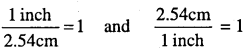∴ 3 inch = 3 × $$\frac{2.54 \mathrm{cm}}{1}$$ ∴ 3 inch = 7.62cm

Question 12.
A jug contains 2L of milk. Calculate the volume of the milk in m3.
We know that 1L = 1000cm3 and 1m = 100cm.
From the equivalence $$\frac{1 \mathrm{m}}{100 \mathrm{cm}}$$ = 1 and $$\frac{100 \mathrm{cm}}{1 \mathrm{m}}$$ = 1
To get m3 from  the above equation
$$\left(\frac{1 \mathrm{m}}{100 \mathrm{cm}}\right)^{3}=1^{3}$$
$$\frac{1 \mathrm{m}^{3}}{(100)^{3} \mathrm{cm}^{3}}=1$$
1m3 = (100)3 cm3
1m3 = 106cm3
2L = 2 × 1000cm3
∴ 2 × 1000cm3 × $$\frac{1 \mathrm{m}^{3}}{10^{6} \mathrm{cm}^{3}}$$ = 2 × 10-3 msup>3

State Law of Chemical Combinations

Question 1.
State law of conservation of mass.
It states that “matter can neither be created nor be destroyed”.
Note: This law was stated by Antoine Lavoisier.

Question 2.
State law of definite proportions or law of definite composition.
This law states that “a given compound always contains exactly the same proportion of elements by weight”.
Note: This law was stated by Proust.

Question 3.
State law of multiple proportions.
This law was stated by Dalton.
This law states that “if two elements can combine to form more than one compound, the masses of one element that combine with a fixed mass of the other element, are in the ratio of small whole numbers”.
Example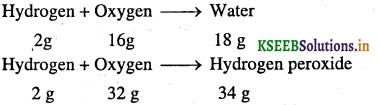Here, the mass of oxygen (16 g and 32 g ) which combine with a fixed mass of hydrogen (2g) bear a simple ratio (16 : 32 or 1 : 2)Question 4.
State Gay Lussac’s law of gaseous volumes
This law states that “when gases combine or produced in a chemical reaction, they do so in a simple ratio by volume provided all gases at same temperature and pressure.
ExampleQuestion 5.
If ten-volume dihydrogen gas reacts with five volumes of dioxygen gas. How many volumes of water vapour would be produced?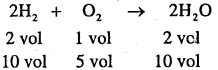∴ 10 volume of H2O will be produced.

Question 6.
This law states that “equal volumes of all gases at the same temperature and pressure contain equal number of molecules”.

Question 7.
Give the postulates of Dalton’s atomic theory.

• Matter consists of indivisible atoms.
• All the atoms.of a given element are identical, and different from those of other elements.
• Con pounds are formed when atoms of different elements combine in a fixed ratio.
• Chemical reactions involve reorganization of atoms.
• Atoms are neither created nor destroyed in a chemical reaction.
Note: Dalton’s theory could explain the laws of chemical combination.

Atomic and Molecular Masses

Question 1.
Define a atomic mass unit (atomic mass).
It is defined as the mass exactly equal to $$\frac { 1 }{ 12 }$$ th the mass of one atom of carbon – 12 isotope.

Question 2.
Name the standard element used to measure atomic mass.
Carbon-12 isotope.

Question 3.
What is the value of 1 amu?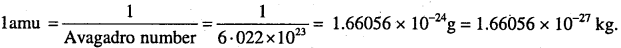Note: Now a days, “amu” has been replaced by ‘u’ which is known as ‘unified mass” .

Question 4.
Calculate the average atomic mass of carbon from the following data.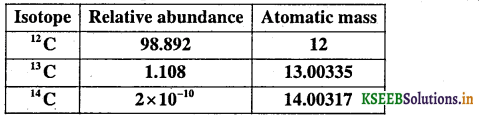Average atomic mass is calculated by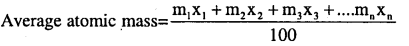Where m1, m2, m3……. are isotope atomic masses x1,x2,x3… are percentage abundance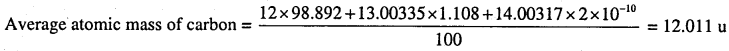Question 5.
Calculate the average atomic mass of chlorine using the following data: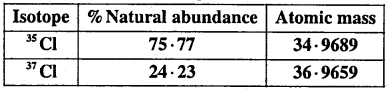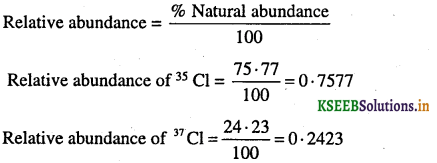Average atomic mass = (0.7577) (34.9689 u) + (0.2423) (36.9659 u)
= 26.4959 + 8.9568 = 35.4527 u.

Question 6.
What is molecular mass? How do you obtain molecular mass from the atomic masses of elements present in the formula?
Molecular mass is the sum of atomic mass of all the elements present in a molecule.
It is obtained by multiplying the atomic mass of each element by the number of its atoms and adding them together.

Question 7.
Calculate the molecular mass of methane
Molecular mass of CH4 = 12.0114 + 4 × 1.0084 = 16.0434 u

Question 8.
Calculate the molecular mass of H2O
Molecular mass of H2O = 2 × 1.0084 + 16.004 = 18.0208 u

Question 9.
Calculate the molecular mass of glucose (C6H12O6) molecule.
Molecular mass of C6H12O6 = 6 × 12.0114 + 12 × 1.0084 + 6 × 16.004 = 180.1932 u

Question 10.
Calculate the molecular mass of CO2
Molecular mass of CO2 = 12.0114 + 2 × 16.004 = 44.0194 u

Question 11.
Formula mass is used in ionic solids instead of molecular mass. Why?
Because ionic solids do not exist as a single entity in the solid state.

Question 12.
Calculate the formula mass of NaCl.
Formula mass of NaCl = 23.0 + 35.5 = 58.5 uMole Concept and Molar Masses

Question 1.
What is the SI unit of amount of substance?
mole (symbol; mol)

Question 2.
Define a mole.
A mole is the amount of substance that contains as many particles or entities as there are atoms in exactly 12g of the carbon-12 isotope.

Question 3.
What is the value of Avogadro’s number?
6.022 × 1023 particles / mole.

Question 4.
How many atoms are present in 12 g of C-12?
6.022 × 1023 atoms.

Question 5.
How many molecules are present in one mole of water?
6.022 × 1023 molecules

Question 6.
Define molar volume.
The volume occupied by one mole of gas at STP is called molar volume.
Note: Molar volume at STP = 22.4 L (273 K, 1 atm)
= 22.7 L (273 K, 1 bar)

Question 7.
What is molar mass?
The mass of one mole of a substance in grams is called its molar mass.
Note: The molar mass in grams is numerically equal to atomic mass or molecular mass or formula mass. Thus,
Molar mass of water = 18.02gmol-1
Molar mass of sodium chloride = 58.5gmol-1

Question 8.
What is the difference between molar mass and molecular mass?
Molar mass is the mass of one mole of a substance expressed in gram per mole where as molecular mass is the mass of one molecule of a substance expressed in unified mass (u).
Example: Molar mass of glucose = 180 g mol-1 . Molecular mass of glucose = 180 uPercentage Composition

Question 1.
How do you calculate the percentage composition of elements in a compounds?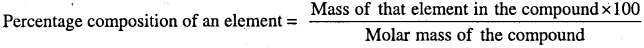Question 2.
Calculate the mass percent of different elements present in sodium sulphate (Na2S04).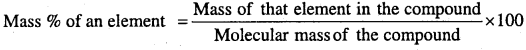Molecular mass of Na2SO4 = 2 × 23.0 + 32.0 + 4 × 16.0 = 142 g mol-1
Mass percent of sodium = $$\frac { 46 }{ 142 }$$ × 100 = 32.39%
Mass percent of sulphur = $$\frac { 32 }{ 142 }$$ × 100 = 22.54%
Mass percent of oxygen = $$\frac { 64 }{ 142 }$$ × 100 = 45.07%

Question 3.
Calculate the percentage composition of hydrogen and oxygen in water.
Molar mass of H2O = 18.02 mol
% Composition of hydrogen = $$\frac{2 \times 1.008 \times 100}{18.02}$$ = 11.18%
% Composition of oxygen = $$\frac{16 \times 100}{18.02}$$ = 88.79%

Question 4.
Calculate the percentage composition of C,H and O in C2H5OH.
Molar mass of C2H5OH = 2 × 12.01 + 6 × 1.008 + 16 = 46.068g mol-1
% of carbon = $$\frac{2 \times 12.01}{46.068}$$ × 100 = 52.14%
% of hydrogen = $$\frac{6 \times 1 \cdot 008}{46.068}$$ × 100 = 13.13%
% of oxygen = $$\frac{16 \times 100}{46.068}$$ = 34.73%

Question 5.
Define empirical formula.
Empirical formula is the simplest formula which represent the simplest whole number ratio of various atoms present in a compound.

Question 6.
What is molecular formula?
It is shows the exact number of different types of atoms present in a molecule of a compound.

Question 7.
Give the simple steps to determine the empirical formula of a compound from the given percentage composition of elements.

• Convert the given % composition of elements into grams.
• Convert them into moles of each element by using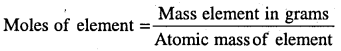• Divide the mole value obtained above by smallest number of them.
• Write the empirical formula by mentioning the numbers after writing the symbols of respective .elements.Question 8.
How do you obtain molecular formula from empirical formula? Or Give the relation between empirical formula and molecular formula.
Molecular formula = (Empirical formula)nQuestion 9.
A compound contains 4.07% Hydrogen, 24.47% Carbon and 71.65% Chlorine. Its molar mass is 98.96 g mol-1. What are its empirical and molecular formula?
Calculation of empirical formula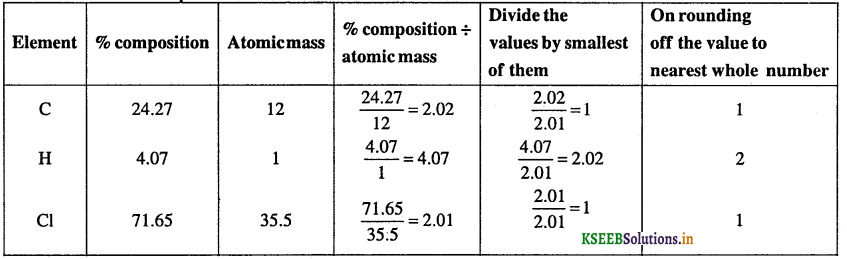Empirical formula is CH2Cl
Empirical formula mass (CH2Cl) = 12 + 2 × 1 + 35.5 = 49.5Molecular formula = (Empirical formula) × n = (CH2Cl)2 = C2H4C12.

Question 10.
A compound with a molecular mass of 34 g/mol is known to contain 5.88% hydrogen and 94.12% oxygen. Find the molecular formula of this compound.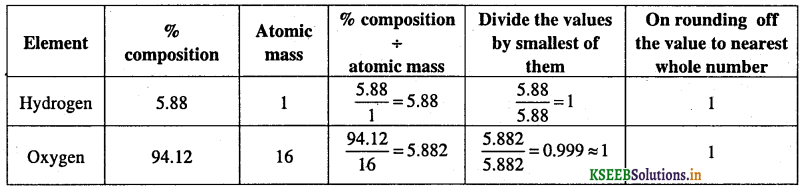Empirical formula = H1O1 = HO
Molecular formula = (Empirical formula)n
Empirical formula mass = 1 × 1 + 1 × 16 = 17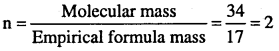∴ Molecular formula = (HO)2 = H2O2

Question 11.
Determine the molecular formula of an oxide of iron in which the mass per cent of iron and oxygen are 69.9 and 30.1 respectively. Given that the molar mass of the oxide is 159.8 g mol-1.
(Atomic mass : Fe = 55.85, O = 16.00 u).
Calculation of empirical formula:Empirical formula = Fe2O3.
Empirical formula mass of Fe2O3 = 2 × 55.85 + 3 × 16.00 = 159.7 g mol-1
Molecular formula = n × Empirical formula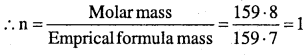∴ M . F = (E . F)n = (Fe2O3)1 = Fe2O3
Hence, molecular formula is same as empirical formula, viz., Fe2O3.Question 12.
A given sample has 30% ethanol by mass, the rest being water. Determine the percentage C, H and O in the given sample.
Molar mass of ethanol = 46 g mol-1
Carbon present in 46 g of ethanol = 2 × 12 = 24g
Carbon present in 30 g of ethanol = $$\frac{24 \times 30}{46}$$ = 15.65 g
Hydrogen present in 30 g ethanol = $$\frac{6 \times 30}{46}$$ = 3.91 g
Oxygen present in 30 g oxygen = $$\frac{16 \times 30}{46}$$ = 10.43 g
In 70% of water,
Mass of hydrogen present = $$\frac{2 \times 70}{18}$$ = 7.78 g
Mass of oxygen present = $$\frac{16 \times 70}{18}$$ = 62.22 g
Percentage of Carbon = 15.65%
Percentage of Hydrogen = 3.91 + 7.78 = 11.69%
Percentage of Oxygen = 10.43 + 62.22 = 72.65%

Stoichiometry and Stoichiometric Calculations

Question 1.
What is stoichiometry?
It deals with the calculation of masses and volumes of the reactants and products involved in a chemical reaction.

Question 2.
What is limiting reagent?
It is defined as the reactant which is completely consumed during the reaction is called limiting reagent.

Question 3.
What is excess reagent?
The reactants that are not completely consumed in a chemical reaction are called excess reagent.

Question 4.
N2 and H2 react with each other to produce ammonia according to the equation
N2(g) + 3H2(g) → 2NH3(g)
(i) Calculate the mass of ammonia produced if 2.0 × 103 g of N2 reacts with 1.0 × 103 g of H2.
(ii) Will any of the two reactants remain unreacted?
(iii) If yes, which one and what would be its mass?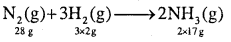28g N2 reacts → 3 × 2g H2
2 × 103 g N2 → x g H2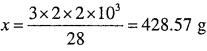Amount of H2 unreacted = 1000 – 428.57 = 571.428 g
∴ Limiting reagent = N2 (∴ N2 is completely reacted).
Limiting reagent should be taken for calculation of amount of products.
28 g N2 produces → 2 × 17 g NH3
2 × 103 g N2 produces → x g NH3
x = $$\frac{2 \times 17 \times 2 \times 10^{3}}{28}$$
x = 2,428.57 g NH3

(ii) Yes.

(iii) 571.42 g unreacted hydrogenQuestion 5.
How many moles of methane are required to produce 22 g CO2(g) after combustion?
According to the chemical equation.∴ 44 g CO2 is obtained from 16 g CH4
1 mole CH4 = 16 g, 1 mole CO2 = 44 g
∴ 1 mole of CH4 → 44 g CO2
x mole CH4 → 22 g CO2
x = $$\frac{1 \times 22}{44}$$ = 0.5 mole CO2
Hence, 0.5 mol of CH4 would be required to produce 22 g CO2.

Question 6.
50.0 kg of N2(g) and 10.0 kg of H2(g) are mixed to produce NH3(g). Calculate the mass of NH3(g) formed. Identify the limiting reagent in the production of NH3.N2(g) + 3H2g ⇌ 2NH3(g)
1 mole of N2 requires = 3 mol H2
Hence, 17.86 × 102 mole of N2 requires = 3 × 7.86 × 102 = 5.36 × 103 mol H2
But, we have only 5.0 × 103mole of H2.
So, H2 is the limiting reagent according to above equation.
3 moles of H2 gives → 2 moles of NH3
5 × 103 mole of H2 gives → x moles of NH3
x = $$\frac{2 \times 5 \times 10^{3}}{3}$$ = 3.3 × 103 mol NH3
But Mass = mole × molecular mass
= 3.30 × 103 × 17 (∵ Molecular mass of NH3 = 17 g mol-1)
= 56.1 × 103g NH3 = 56.3 kgNH3

Question 7.
30 g Magnesium and 30 g of O2 react according to the equation 2Mg + O2 → 2MgO.
(i) What is the limiting reagent and
(ii) What is the composition of the residual mixture.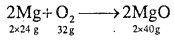2 × 24g Mg reacts → 32 g O2
30 g Mg reacts → x O2
x = $$\frac{32 \times 30}{2 \times 24}$$ = 20 g O2 reacted
∴ Amount of O2 unreacted = 30 – 20 = 10g
(i) Limiting reagent = Mg (completely reacted)
(ii) 2 × 24 g Mg produces → 2 × 40 g MgO
30 g Mg produces → x g
x = $$\frac{2 \times 40 \times 30}{2 \times 24}$$ = 50 g
∴ Residual mixture contains 10 g unreacted O2 and 50 g MgOQuestion 8.
Calculate the amount of water (g) produced by the combustion of 16 g of methane.
The balanced equation for combination of methane is:From the above equation, 1 mol of CH4 gives 2 mol of H2O.
1 mole of CH4 = 16 g CH4
1 mol H2O = 18g H2O
∴ 16 g CH4 gives 2 × 18 g waler.
Hence 2 mol H2O = 2 × 18gH2O = 36g H2O.

Question 9.
Ammonia gas and hydrogen chloride gas combine to make ammonium chloride. What volume of ammonia at STP is needed to react with 47.7 litres of hydrogen chloride at STP?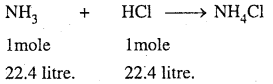22.4 litre of HCl combines ……… 22.41itre of NH3
47.7 litre of HCl combines ……… x litre of NH3
x = $$\frac{47.7 \times 22.4}{22.4}$$ = 47.7lit of NH3 to form ammonium chloride.

Question 10.
Calculate the amount of carbon dioxide that could be produced when
(i) 1 mole of carbon is burnt in air.
(ii) 1 mole of carbon is burnt in 16 g of dioxygen,
(iii) 2 moles of carbon are burnt in 16 g of dioxygen.
(i) The balanced equation for the combustion of carbon in dioxygen or air is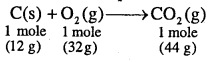(ii) According to the above equation
As only 16 g of dioxygen is available, it is the limiting reagent
32 g dioxygen produces → 44 g CO2
16 g dioxygen produces → xg CO2
x = $$\frac{44 \times 16}{32}$$ = 22g

(iii) Here again, .dioxygen is the limiting reagent
Therefore, CO2 produced from 16 g dioxygen = $$\frac { 44 }{ 32 }$$ × 16 = 22g

Question 11.
In three moles of ethane (C2H6), calculate the following:
(i) Number of moles of carbon atoms
(ii) Number of moles of hydrogen atoms
(iii) Number of molecules of ethane.
(i) 1 mole of C2H6 contains 2 moles of carbon atoms
∴ 3 moles of C2H6 will contain 3 × 2 = 6 moles of carbon atoms.

(ii) 1 mole of C2H6 contains 6 moles of hydrogen atoms
∴ 3 moles of C2H6 will contain 3 × 6 = 18 moles of hydrogen atoms.

(iii) 1 mole of C2H6 contains Avogadro’s number, i.e., 6.02 × 1023 molecules
∴ 3 moles of C2H6 will contain,
= 3 × 6.02 × 1023 = 18.06 × 1023 molecules of ethaneQuestion 12.
In the reaction, A + B2 → AB2 identify the limiting reagent, if any, in the following mixtures:
(i) 300 atoms of A + 200 molecules of B
(ii) 2 mol A + 3 mol B
(iii) 100 atoms of A + 100 molecules of B
(iv) 5 mol A + 2.5 mol B
(v) 2.5 mol A + 5 mol B
(i) According to the given reaction, 1 atom of A reacts with 1 molecule of B. .’. 200 molecules of B will react with 200 atoms of A and 100 atoms of A will be left unreacted. Hence, B is the limiting reagent while A is the excess reagent.

(ii) According to the given reaction, 1 mol of A reacts with 1 mol of B, 2 mol of A will react with 2 mol of B. Hence, A is the limiting reactant.

(iii) No limiting reagent.

(iv) 2.5 mol of B will react with 2.5 mol A as per the given equation. Hence, B is the limiting reagent.

(v) 2.5 mol of A will react with 2.5 mol of B. Hence, A is the limiting reagent.

Question 13.
Dinitrogen and dihydrogen react with each other to produce ammonia according to the following chemical equation: N2 (g) + 3H2 (g) → 2 NH3 (g)
(i) Calculate the mass of ammonia produced if 2.00 × 103 g dinitrogen reacts with 1.00 × 103 g dihydrogen,
(ii) Will any of the two reactants remain unreacted?
(iii) If yes, which one and what would be its mass?
(i) According to given equation, 28 g of N2 reacts with 3 mol of H2, i.e., 6 g of H2
∴ 2000 g of N2 will react with H2 = $$\frac { 6 }{ 28 }$$ × 2000g = 428.6 g.
Amount of H2 given = 1000 g
Thus, N2 is the limiting reagent while H2 is the excess reagent.
As 28 g of N2 produce NH3 = 2 × 17 = 34 g
∴ 2000 g of N2 will produce NH3 = $$\frac { 34 }{ 28 }$$ × 2000g = 2428.57 g

(ii) H2 will remain unreacted.
(iii) Mass of H2 left unreacted = 1000 g – 428.6 g = 571.4 g

Reactions in Solutions

Question 1.
Define mass percent.
It is defined as the mass of solute present in 100g of the solution. It is calculated by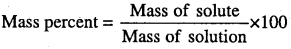Question 2.
A solution is prepared by adding 2 g of a substance A to 18 g of water. Calculate mass percent of the solute.
Mass percent of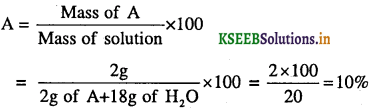Question 3.
Define molarity. Write the expression to calculate the molarity of solution for the given mass of solute and volume of the solution.
It is defined as the number of moles of solute present in 1L or 1000cm3 of the solution. It is calculated by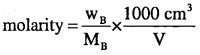Where WB = mass of solute, MB = molar mass of solute ‘
V = volume of the solution.Question 4.
Calculate the molarity of sucrose (C12H22O11) in mol L-1 if 10g of sucrose is dissolved in enough water to make a final volume of 500ml.Question 5.
Calculate the molarity of NaOH in the solution prepared by dissolving 4 g of NaOH in enough water to form 250 mL of the solution.
Mass of solute (NaOH) = 4 g
Molar mass of solute (NaOH) = (23 + 16 + 1) = 40 g mol-1
Volume of solution = 250 mlQuestion 6.
Calculate the concentration of nitric acid in moles per litre in a sample which has a density 1.41g ml-1 and mass percentage of nitric acid in it will be 69%.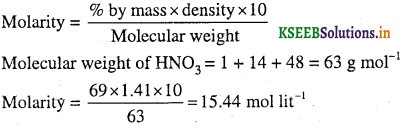Question 7.
How much volume of 18M and 4M sulphuric acid must be mixed to get one litre of 8M sulphuric acid?
Consider the volume of 18M H2SO4 solution = x L
The volume of 4M H2SO4 to be mixed = (1 – x) L
Mixing above two acid solutions we obtain one litre of 8M H2SO4
Calculation to find x:
(M1V1 + M2V2) = (M3V3)
Mixing solution = required solution
18x + 4(1 – x) = 8 × 1
18x + 4 – 4x = 8
14 x = 4
∴ x = $$\frac { 4 }{ 14 }$$ = 0.2857L = 285.7 ml .
x = 0.2857 lit
Volume of 18M H2SO4 solution = 0.2857 lit = 285.7 ml
Volume of 4M H2SO4 solution = 1000 – 285.7 = 714.3 ml

Question 8.
Define molality. Write the expression to calculate molality of the solution for the given mass of solute and solvent.
It is defined as the number of moles of solute present in 1 kg or 1000g of solvent. It is calculated by the equation,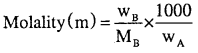Question 9.
The density of 3 M solution of NaCl is 1.25 g mol-1. Calculate molality of the solution.
Molar mass of NaCl = 23 + 35.5 = 58.5 g mol-1
3 M means that 3 mole of solute dissolved in 1000 ml of solution.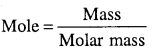Mass of solute (NaCl) = Molar mass × moles of NaCl = 58.5 × 3 = 175.5 g .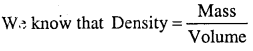So Mass of solution = Volume of Solution x Density = 1000 × 1.25 = 1250 g
Mass of solvent = Mass of solution – Mass of solute = 1250 – 175.5 = 1074.5Question 10.
Calculate the molality of a solution when 1.5 moles is added to 675 ml of solvent (assume density of solvent = lg/ml)Question 11.
A sample of drinking water was found to be severely contaminated with chloroform, CHCl3, supposed to be carcinogenic. The level of contamination was 15 ppm (by mass).
(j) Express this in percent by mass.
(ii) Determine the molality of chloroform in the water sample.
(i) 15 ppm means 15 parts in million (106) parts
∴ % by mass = $$\frac{15}{10^{6}}$$ × 100 =15 × 10-4 =1.5 × 10-3%

(ii) Molar mass of chloroform (CHCl3) = 12 + 1 + 3 × 35.5 = 119.5 g mol-1
100 g of the sample contain chloroform = 1.5 × 10-3 g
∴ 1000 g (1 kg) of the sample will contain chloroform = $$\frac{1 \cdot 5 \times 10^{-3}}{100}$$ × 1000 = 1.5 × 10-2 g
Moles of chloroform ¡n 1 kg (molaiity) = $$\frac{1 \cdot 5 \times 10^{-2}}{119 \cdot 5}$$ = 1.255 × 10-4 m

Question 12.
Define mole fraction.